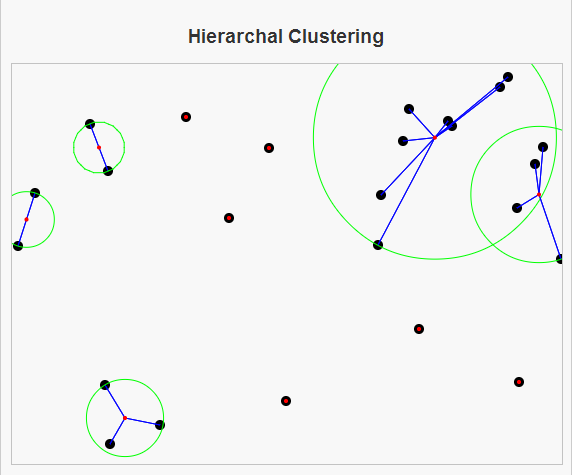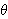# Hierarchical ClusteringHierarchical Clustering algorithms give a nice introduction for computer science students to unsupervised machine learning. I say this because the bottom-up approach to Hierarchical clustering (which I have implemented here) is very similar to Kruskal’s algorithm for finding the minimum spanning tree of a graph.

In Kruskal’s algorithm, we begin by creating a forest, or a set of trees where each node is its own tree. The algorithm then selects the two trees that are closest together (closest being defined as the minimum cost edge between two distinct trees) and merges those trees together. This process of merging the closest two trees is then repeated until there is only one tree remaining, which is a minimum spanning tree of the graph.

Similarly, bottom-up hierarchical clustering of a group of points begins by saying that each point is its own cluster. Then the clusters are compared to one another to check if two clusters will be merged into one. Generally, there will be some stopping criteria,, saying that we do not want to merge two clusters together if their distance is greater than. So if the minimum distance between two clusters is less thanwe will proceed as in Kruskal’s algorithm by merging these two clusters together into one cluster. We repeat this process of merging the closest two clusters together until we find that the minimum distance between two clusters is greater than or equal to, in which case we can stop and the result is a partition of our data set into distinct clusters.

Hierarchical clustering is comparable to K-Means Clustering. Here are some differences between the two approaches:

1. K-Means Clustering requires an initial number of desired clusters, while Hierarchical clustering does not.
2. A run of K-Means Clustering will always give K clusters, whereas Hierarchical Clustering can give more or less, depending on our tolerance.
3. K-Means can undo previous mistakes (assignments of an element to the wrong cluster), while Hierarchical Clustering cannot.

So, here is a link to my page on Hierarchical Clustering. Hope you enjoy.

# K-Means ClusteringI have now uploaded my K-Means Clustering Script. The script generates a set of random numbers (as ordered pairs) and asks the user how many clusters we should divide the numbers into and a maximum number of iterations to go through before we stop.

One of the largest problems that we face today is understanding data. Before we even get to the point of trying to interpret what the data means and making decisions based on that data there is often a problem with the general amount of data. Clustering algorithms seek to solve this problem by defining some notion of similarity and using that notion to group the data into sets or ‘clusters’, where two elements belong to the same cluster if they are considered similar. Once elements are placed into clusters, we can analyze this (generally smaller) set of clusters instead of the entire data set, which should help in understanding the data.

Finding an exact solution to this problem is computationally difficult. Instead, we can approximate a solution rather quickly using the k-means clustering algorithm. This algorithm attempts to separate a given data set into a user specified (k) number of groups. The k signifies the number of clusters that we will generate. The algorithm works by initially selecting k elements of the data to serve as the “center” of each cluster. Every element of the data is then compared to each cluster center and assigned to the cluster with the closest cluster center. Once every element in the data is assigned to a cluster, the cluster centers may have changed. So the next step is to measure the elements inside each cluster and determine the new cluster center. The process of assigning elements to (new) clusters and determining (new) cluster centers is repeated until either no element changes cluster or we have reached some maximum number of iteration that the user specifies that they do not want to exceed.

K-Means Clustering can be thought of as an algorithm in the area of unsupervised machine learning. Machine learning is a field of artificial intelligence that focuses on computer programs that have the ability to learn without being explicitly programmed. Unsupervised machine learning seeks to make interpret data without any knowledge of what a “correct” interpretation is. In comparison, supervised machine learning algorithms are useful for data that has been separated into categories. These algorithms generally divide the data into a training set and a test set and seek to produce a function that agrees with the results on the training set.